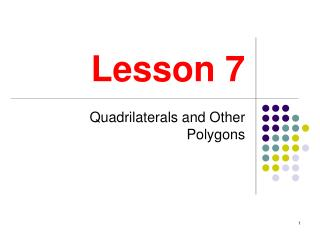DownloadDownload PresentationLesson 7

# Lesson 7

Download Presentation## Lesson 7

- - - - - - - - - - - - - - - - - - - - - - - - - - - E N D - - - - - - - - - - - - - - - - - - - - - - - - - - -
##### Presentation Transcript

1. Lesson 7 Quadrilaterals and Other Polygons

2. Quadrilaterals • A quadrilateral is a four-sided figure like the one shown. The sides are line segments connected together at their endpoints, called the vertices (plural of vertex) of the quadrilateral. • We call this quadrilateral ABCD or BADC or CDAB etc. (We start with a vertex and then proceed around the quadrilateral, either clockwise or counterclockwise.) • The line segments shown are called diagonals. D C A B

3. Rectangles • A rectangle is a quadrilateral with four right angles. • The opposite sides of a rectangle are congruent and parallel. • Each diagonal of a rectangle divides the rectangle into two congruent triangles. • The diagonals bisect each other and are congruen to each other. • The width of a rectangle is the common length of one pair of opposite sides. • The length of a rectangle is the common length of another pair of opposite sides. • Instead of width and length, we sometimes use base and height. D C A B

4. Example • The length of a rectangle is 12 and the width is 5. What is the length of one of its diagonals? • It’s a good idea to draw a picture. • The diagonal is the hypotenuse of a right triangle whose legs measure 5 and 12. So, we use the Pythagorean Theorem: c 12 5

5. Squares • A square is a rectangle in which all four sides are congruent. • Each diagonal divides the square into two 45-45-90 triangles. Both diagonals together divide the square into four 45-45-90 triangles. • If a side of the square measures then each diagonal measures • The diagonals bisect each other and are congruent to each other.

6. D E P C A F G B Example • In the figure, ABCD and AFDE are squares. • If then what is • First note that is a 45-45-90 triangle. So, • So, • But So, too. • So,

7. Parallelograms • A parallelogram is a quadrilateral in which both pairs of opposite sides are parallel. • Each pair of opposite sides in a parallelogram are not only parallel, but congruent as well. • Each diagonal divides the parallelogram into two congruent triangles. • The diagonals of a parallelogram bisect each other. • Opposite angles of a parallelogram are congruent. • Consecutive angles of a parallelogram are supplementary.

8. Rhombi • A rhombus (plural rhombi) is a parallelogram with four congruent sides. • Since a rhombus is a parallelogram, it has all the properties a parallelogram has. • In addition, the diagonals of a rhombus are perpendicular to each other and they divide the rhombus into four congruent right triangles.

9. Trapezoids • A trapezoid is a quadrilateral such that one pair of opposite sides is parallel. • The parallel sides are called the bases of the trapezoid. • The nonparallel sides are called the legs of the trapezoid. • If the legs of a trapezoid are congruent, the the trapezoid is called isosceles. • In an isosceles trapezoid, the diagonals bisect each other.

10. Kites • A kite is a quadrilateral that has two pairs of congruent sides. The congruent sides are consecutive, not opposite. • A kite has one pair of opposite angles that are congruent. • One of the diagonals bisects the other. • The diagonals are perpendicular to each other.

11. Polygons • A polygon is a closed figure, like the one shown, made up of joining line segments together at their endpoints. • Triangles and quadrilaterals are examples of polygons. • A polygon with 5 sides is called a pentagon. • A polygon with 6 sides is called a hexagon. • A polygon with 7 sides is called a heptagon. • A polygon with 8 sides is called an octagon. • A polygon with 9 sides is called a nonagon. • A polygon with 10 sides is called a decagon. • A regular polygon is a polygon in which all of its sides are congruent and all of its interior angles are congruent.

12. Interior Angles of a Polygon • If a polygon has n sides, then the sum of the measures of its n angles is • Each interior angle of a regular polygon with n sides measures

13. 1 Exterior Angles of a Polygon • An exterior angle of a polygon is formed by a side of the polygon and an extension of an adjacent side. • in the figure is an exterior angle of a regular hexagon. • An exterior angle is the supplement of an interior angle. • In any polygon if we draw one exterior angle at each vertex then the sum of the measures of these exterior angles is

14. Diagonals of a Polygon • A diagonal of a polygon is a line segment connecting two non-consecutive vertices. • For example, a triangle has no diagonals and a quadrilateral has two diagonals. • In general, if a polygon has n sides, then it has diagonals.